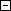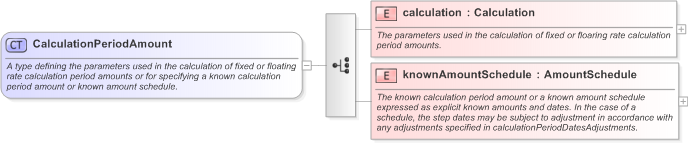Definition Type: ComplexType Name: CalculationPeriodAmount Namespace: http://www.fpml.org/FpML-5/transparency Containing Schema: fpml-ird-5-9.xsd Abstract Documentation: A type defining the parameters used in the calculation of fixed or floating rate calculation period amounts or for specifying a known calculation period amount or known amount schedule.XSD Schema Diagram:XSD Schema Code: `````` A type defining the parameters used in the calculation of fixed or floating rate calculation period amounts or for specifying a known calculation period amount or known amount schedule. The parameters used in the calculation of fixed or floaring rate calculation period amounts. The known calculation period amount or a known amount schedule expressed as explicit known amounts and dates. In the case of a schedule, the step dates may be subject to adjustment in accordance with any adjustments specified in calculationPeriodDatesAdjustments. ``````Child Elements:
 Name Type Min Occurs Max Occurs calculation nsA:calculation (1) (1) knownAmountSchedule nsA:knownAmountSchedule (1) (1)Derivation Tree: CalculationPeriodAmountReferences: nsA:calculationPeriodAmountComments: Please enable JavaScript to view the comments powered by Disqus. blog comments powered by Disqus